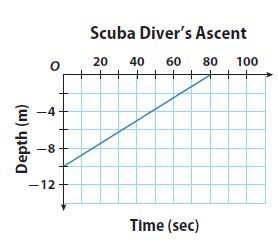Homework Explained - Math Practice 101Dear guest, you are not a registered member. As a guest, you only have read-only access to our books, tests and other practice materials.

As a registered member you can:

Registration is free and doesn't require any type of payment information. Click here to Register.
Go to page:
Chapter 5: Writing Linear Equations; Lesson 1: Writing Linear Equations from Situations and Graphs

Independent Practice

• Question 5

A dragonfly can beat its wings 30 times per second. Write an equation in slope-intercept form that shows the relationship between flying time in seconds and the number of times the dragonfly beats its wings

• Type below:
• Question 6

A balloon is released from the top of a platform that is 50 meters tall. The balloon rises at the rate of 4 meters per second. Write an equation in slope-intercept form that tells the height of the balloon above the ground after a given number of seconds.

• Type below:

The graph shows a scuba diver’s ascent over time.• Question 7

Use the graph to find the slope of the line. Tell what the slope means in this context.

•  Slope = meters per second
• Question 8

Identify the y-intercept. Tell what the y-intercept means in this context.

•  y-intercept= meters
• Question 9

Write an equation in slope-intercept form that represents the diver’s depth over time.

• Type below:
• Question 10

The formula for converting Celsius temperatures to Fahrenheit temperatures is a linear equation. Water freezes at $$0^{o}$$C, or $$32^{o}$$F, and it boils at $$100 ^{o}$$C, or $$212^{o}$$F. Find the slope and y-intercept for a graph that gives degrees Celsius on the horizontal axis and degrees Fahrenheit on the vertical axis. Then write an equation in slope-intercept form that converts degrees Celsius into degrees Fahrenheit.

• Type below:
• Question 11

The cost of renting a sailboat at a lake is $20 per hour plus$12 for lifejackets. Write an equation in slope-intercept form that can be used to calculate the total amount you would pay for using this sailboat.

• Type below:

Yes, email page to my online tutor. (if you didn't add a tutor yet, you can add one here)